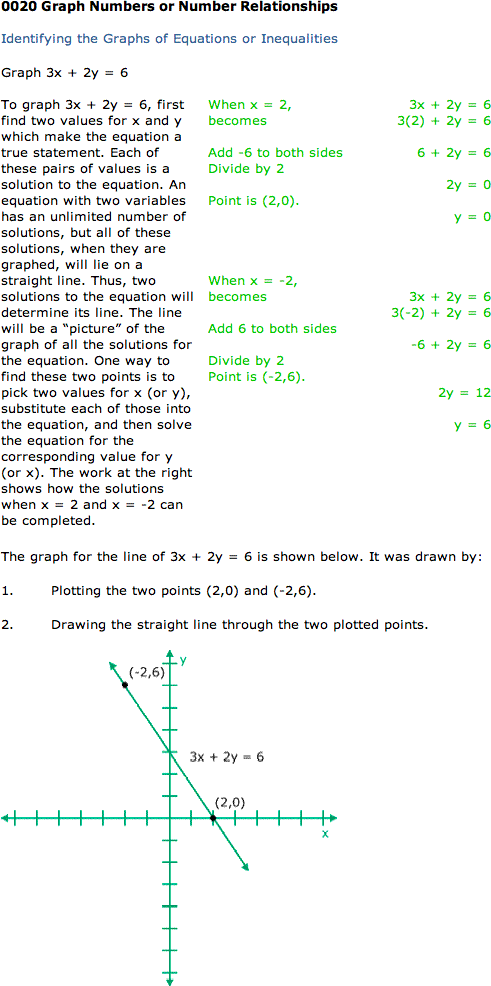Welcome, Student Name | Student Demo | Buy Now

 Contents Mathematics (98)0016 Number Concepts and Computation Skills0017 Word Problems Involving Integers, Fractions, or Decimals0018 Interpret Information from a Graph, Table, or Chart0019 Probability and Statistics0020 Graph Numbers or Number RelationshipsPoints or Graphs of Sets of Ordered PairsGraphs of Equations or InequalitiesSlopes and Intercepts of LinesDirect and Inverse Variation0021 One- and Two-Variable Equations0022 Word Problems Involving One and Two Variables0023 Algebraic Expressions0024 Problems Involving Geometric Figures0025 Apply Reasoning SkillsPractice Math Tests Main Menu Exit Student Demo Buy Now for \$39.95 and begin using the entire course in less than two minutes